Win up to 100% scholarship on Aakash BYJU'S JEE/NEET courses with ABNAT Win up to 100% scholarship on Aakash BYJU'S JEE/NEET courses with ABNAT

# JEE Main 2020 Physics Paper With Solutions Jan 7 Shift 1

JEE Main 2020 was conducted in the first week of January (Jan 7th). It is an examination conducted for candidates willing to join reputed organizations like IIT, NIT etc. The examination was conducted by the national testing authority in various centres across the country. Candidates can find the answers to all the questions asked in the Physics paper held on 7 January 2020 JEE Main shift 1 in this page.

## JEE Main 2020 Physics Paper With Solutions Jan 7 Shift 1

January 7 Shift 1 – Physics
1. A polarizer-analyzer set is adjusted such that the intensity of light coming out of the analyzer is just 10 % of the original intensity. Assuming that the polarizer-analyzer set does not absorb any light, the angle by which the analyser need to be rotated further to reduce the output intensity to be zero is
a. 45o
b. 90o
c. 71.6o
d. 18.40

Intensity after polarisation through polaroid = Iocos2φ

So, 0.1Io = Iocos2φ

⇒cosφ = √0.1

⇒cosφ = 0.316

Since, cos φ < cos 45o therefore, φ > 45o If the light is passing at 90o from the plane of polaroid, than its intensity will be zero.

Then, θ = 90o − φ therefore, θ will be less than 45o. So, the only option matching is option d which is 18.4o

2. Which of the following gives reversible operation?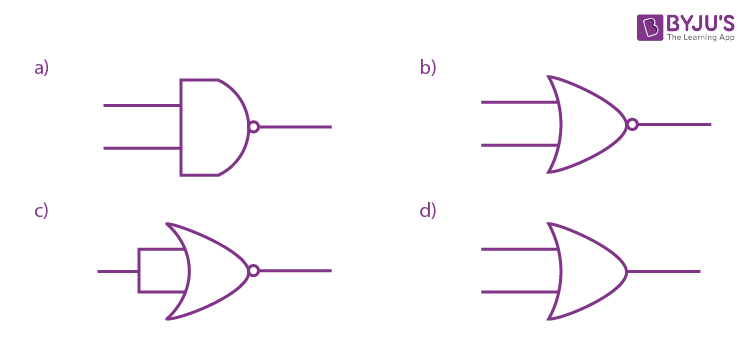Since, there is only one input hence the operation is reversible.

3. A 60 HP electric motor lifts an elevator with a maximum total load capacity of 2000 kg. If the frictional force on the elevator is 4000 N, the speed of the elevator at full load is close to (Given 1 HP = 746 W, g = 10 m/s2)
a. 1.5 m/s
b. 1.7 m/s
c. 2.0 m/s
d. 1.9 m/s

P = Fv + Mgv

Applied power = 4000 × v + 20000 v

60 × 246 = 4000 v + 20000 v

v = 1.865

v = 1.9 m/s Rounding it off.

4. A long solenoid of radius R carries a time (t) dependent current I(t) = I0t(1 − t). A ring of radius 2R is placed coaxially near its middle. During the time instant 0 ≤ t ≤ 1, the induced current (IR) and the induced EMF (VR) in the ring changes as:
a. Direction of IR remains unchanged and VR is maximum at t = 0.5
b. Direction of IR remains unchanged and VR is zero at t = 0.25
c. At t = 0.5 direction of IR reverses and VR is zero
d. At t = 0.25 direction of IR reverses and VR is maximum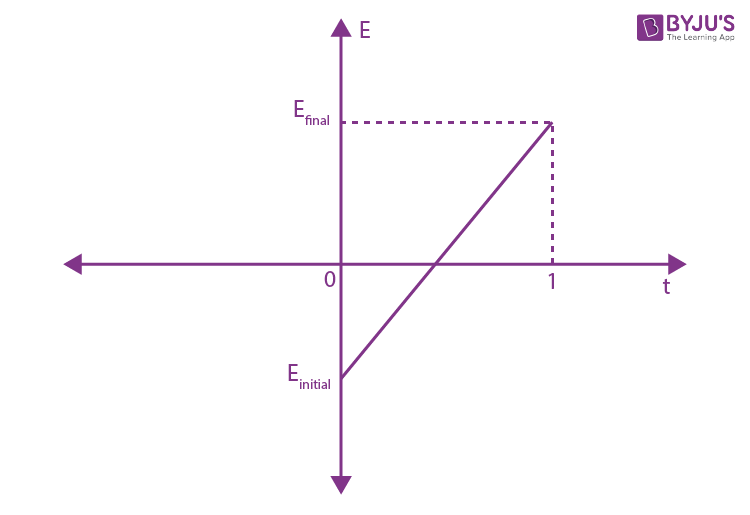Field due to solenoid near the middle = µoNI

Flux, φ = BA

Where (A = πR2)

= µoNIot(1 − t)πR2

E = − dφ/dt [By Lenz’s law]

$$\begin{array}{l}E = -\frac{d}{dt}(\mu _{0}NI_{0}t(1-t)\pi R^{2})\end{array}$$
$$\begin{array}{l}E = -\mu _{0}NI_{0}\pi R^{2}\frac{d}{dt}(t(1-t))\end{array}$$

E = −πµoIoNR2(1 − 2t)

Current will change its direction when EMF will be zero

= (1- 2t) = 0

So, t = 0.5 sec

5. Two moles of an ideal gas with Cp/Cv = 5/3 are mixed with 3 moles of another ideal gas with Cp/Cv = 4/3. The value of Cp/Cv for the mixture is
a. 1.47
b. 1.4
c. 1.42
d. 1.50

For γ = Cp/Cv = 5/3

Using formula Cp = Rγ/γ-1

Cv = R/γ-1

Cp = 5R/2

Cv = 3R/2

Similarly for 2nd gas having γ = Cp/Cv =4/3

Cp = 4R

Cv = 3R

Now γ of mixture =

$$\begin{array}{l}\frac{n_{1}C_{p1}+ n_{2}C_{p2}}{n_{1}C_{v1}+ n_{2}C_{v2}}\end{array}$$

Given that n1 = 2 and n2 = 3

$$\begin{array}{l}\gamma = \frac{2\times \frac{5R}{2}+3\times4R}{2\times \frac{3R}{2}+3\times 3R}\end{array}$$

= 17/12 = 1.42

6. Consider a circular coil of wire carrying current I, forming a magnetic dipole. The magnetic flux through an infinite plane that contains the circular coil and excluding the circular coil area is given by φi The magnetic flux through the area of the circular coil area is given by φ0.

Which of the following option is correct?

a. φi= −φo
b. φio
c. φio
d. φio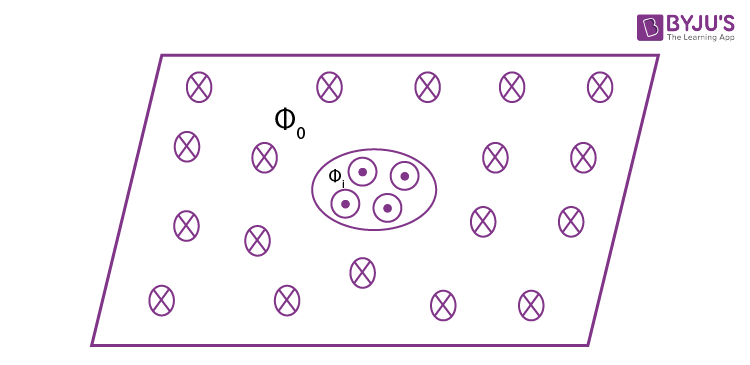As magnetic field line of ring will form close loop.

∴ φ1 = −φ0 (because the magnetic field lines going inside is equal to the magnetic field lines coming out.)

7. The current (in A) flowing through 1 Ω resistor in the following circuit is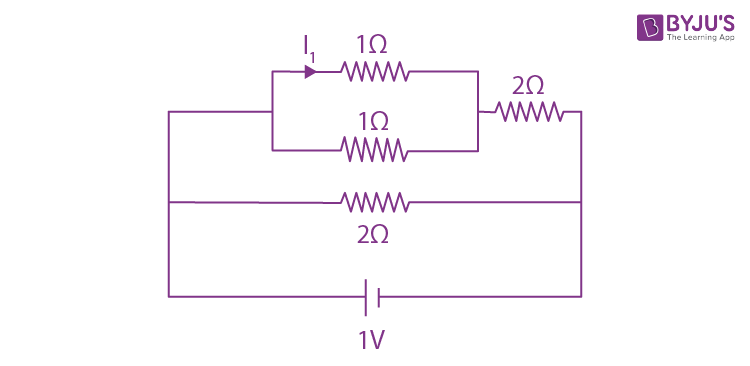a. 0.40 A
b. 0.25 A
c. 0.20 A
d. 0.5 A

Net resistance across CD = 1/2 Ω

Net resistance across BE = 2 + (1/2) = (5/2) Ω

Net resistance across BE = [(5/2) x 2] / [(5/2) x 2] = (10/9) Ω

Total current in circuit = V/R = 9/10 A

In the given circuit,voltage across BE = voltage across BF = 1 V

Current across BE = VBE/R = (2/5) A

Current across CD and DE will be same which is 2/5 A.

Now, current across any 1 Ω resistor will be same and given by = I = (1/2) × (2/5) = 1/5 = 0.20 A

8. Two infinite planes each with uniform surface charge density +σ C/m2 are kept in such a way that the angle between them is 30o. The electric field in the region shown between them is given by: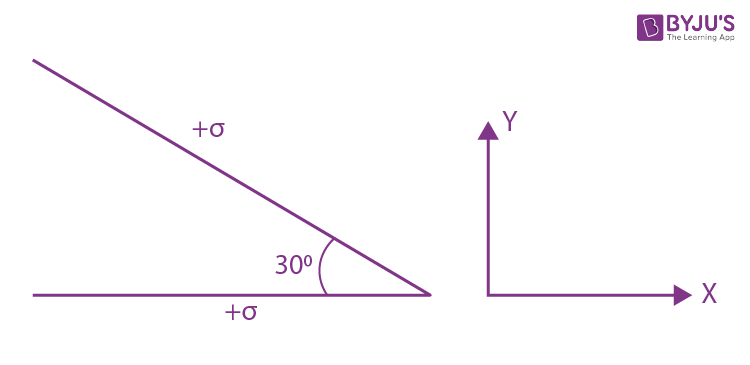a.

$$\begin{array}{l}\frac{\sigma }{2\epsilon _{0}}\left [ (1-\frac{\sqrt{3}}{2})\hat{y}-\frac{1}{2}\hat{x}) \right ]\end{array}$$

b.
$$\begin{array}{l}\frac{\sigma }{2\epsilon _{0}}\left [ (1+\frac{\sqrt{3}}{2})\hat{y}-\frac{1}{2}\hat{x}) \right ]\end{array}$$

c.
$$\begin{array}{l}\frac{\sigma }{2\epsilon _{0}}\left [ (1-\frac{\sqrt{3}}{2})\hat{y}+\frac{1}{2}\hat{x}) \right ]\end{array}$$

d.
$$\begin{array}{l}\frac{\sigma }{2\epsilon _{0}}\left [ (1+\frac{\sqrt{3}}{2})\hat{y}+\frac{1}{2}\hat{x}) \right ]\end{array}$$

Field due to single plate =

$$\begin{array}{l}\frac{\sigma }{2\epsilon _{0}} =\left [ \vec{E_{1}} \right ]=\left [ \vec{E_{2}} \right ]\end{array}$$
$$\begin{array}{l}\vec{E}= \vec{E_{1}}+\vec{E_{2}}\end{array}$$
$$\begin{array}{l}\frac{\sigma }{2\epsilon _{0}}cos 30^{0}(-j)+\frac{\sigma }{2\epsilon _{0}}sin 30^{0}(-i)+\frac{\sigma }{2\epsilon _{0}}(j)\end{array}$$
$$\begin{array}{l}\frac{\sigma }{2\epsilon _{0}}\left [ (1-\frac{\sqrt{3}}{2})\hat{j}-\frac{1}{2}\hat{i} \right ]\end{array}$$
$$\begin{array}{l}\frac{\sigma }{2\epsilon _{0}}\left [ (1-\frac{\sqrt{3}}{2})\hat{y}-\frac{1}{2}\hat{x}) \right ]\end{array}$$

9. If the magnetic field in a plane electromagnetic wave is given by B˙ = 3 × 10−8 sin(1.6 × 103x + 48 × 1010 t)ˆj T then what will be expression for electric field?
a. E˙ = 3 × 10−8sin(1.6 × 103x + 48 × 1010t)ˆi V/m
b. E˙ = 3 × 10−8sin(1.6 × 103x + 48 × 1010t)ˆj V/m
c. E˙ = 60 sin(1.6 × 103x + 48 × 1010t)kˆ V/m
d. E˙ = 9 sin(1.6 × 103x + 48 × 1010t)kˆ V/m

We know that,

$$\begin{array}{l}\left | \frac{E_{0}}{B_{0}} \right |=c\end{array}$$

B0 = 3 × 10−8

=⇒ E0 = B0 × c = 3 × 10−8 × 3 × 108

= 9 N/C

∴ E = Eosin(ωt − kx + φ)kˆ = 9 sin(ωt − kx + φ)kˆ

E˙ = 9 sin (1.6 × 103x + 48 × 1010t)kˆ V/m

10. The time period of revolution of electron in its ground state orbit in a hydrogen atom is 1.6 × 10−16s. The frequency of revolution of the electron in its first excited state (in s−1) is
a. 6.2 × 1015
b. 7.8 × 1014
c. 1.6 × 1014
d. 5.6 × 1012

Time period is proportional to n3 /Z2

Let T1 be the time period in ground state and T2 be the time period in its first excited state.

T1 = n3 /22

(Where, n = excitation level and 2 is atomic no.)

(T1/T2) = (n1/n2)3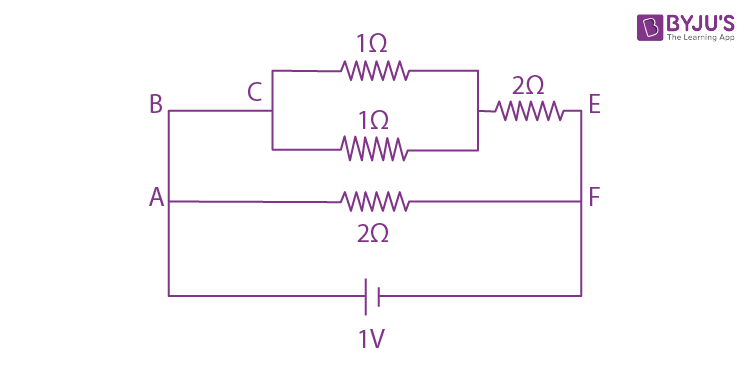Given,

T1 = 1.6 × 10−16s

So,

(1.6 × 10−16) / T2= (1/2)3

T2 = 12.8 x 10-16 s

Frequency is given by f = 1/T

f = 1/ (12.8 x 10-16) Hz

f = 7.8128 × 1014 Hz

11. A LCR circuit behaves like a damped harmonic oscillator. Comparing it with a physical spring-mass damped oscillator having damping constant ’b’, the correct equivalence will be
a. L ↔1/b, C ↔1/m, R ↔1/k
b. L ↔ k, C ↔ b, R ↔ m
c. L ↔ m, C ↔ k, R ↔ b
d. L ↔ m, C ↔ 1/k , R ↔ b

For damped oscillator by Newton’s second law

− kx − bv = ma

kx + bv + ma = 0

kx + b(dx/dt) + m(d2x/dt2) =0

For LCR circuit by KVL

− IR − L (dI/dt)–q/c = 0

⇒ IR + L (dI/dt) + q/c = 0

⇒ q/c + R(dq/dt) + L(d2q/dt2) = 0

By comparing

R ⇒ b

c ⇒ 1/k

m ⇒ L

12. Visible light of wavelength 6000 × 10−8 cm falls normally on a single slit and produces a diffraction pattern. It is found that the second diffraction minima is at 60o from the central maxima. If the first minimum is produced at θ1, then θ1 is close to
a. 20o
b. 45o
c. 30o
d. 25o

For single slit diffraction experiment: Angle of minima are given by

sin θn = nλ/d (sin θn ≠ θn as θ is large )

sin θ2 = sin 60 = √3/2 = 2λ/ d = (2 × 6000 × 10−10)/d ……1

sin θ1 = λ/d= (6000 x 10−10)/d ……2

Dividing (1) and (2)

$$\begin{array}{l}\frac{\frac{\sqrt{3}}{2}}{sin \theta _{1}} = 2\end{array}$$

⇒ sin θ1 = √3/4 = 0.43

As, the value is coming less than 30o the only available option are 20o and 25o but by using approximation we get θ1 = 25

13. The radius of gyration of a uniform rod of length l about an axis passing through a point l/4 away from the center of the rod, and perpendicular to it, is
a. √ (7/48)l
b. √ (3/8) l
c. (1/4) l
d. (1/8) l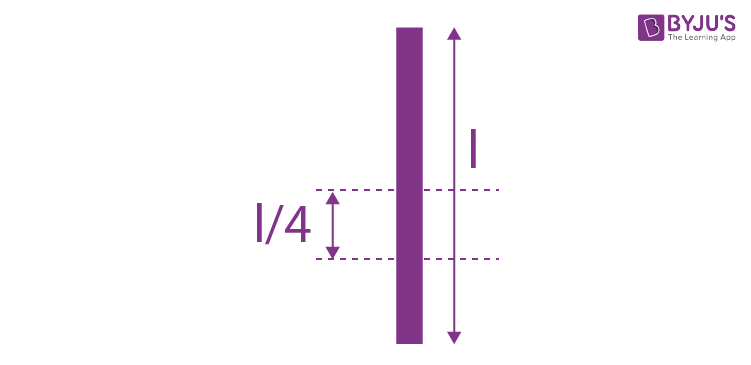Moment of inertia of rod about axis perpendicular to it passing through its centre is given by

I = Ml2/12 + M(l/4)2

I = (3Ml2 + 4Ml2)/48

Now, comparing with I = Mk2 where k is the radius of gyration

k=√7l2/48

k=l√7/48

14. A satellite of mass m is launched vertically upward with an initial speed u from the surface of the earth. After it reaches height R(R = radius of earth), it ejects a rocket of mass m/10 so that subsequently the satellite moves in a circular orbit. The kinetic energy of the rocket is (G = gravitational constant; M is the mass of earth)
a.
$$\begin{array}{l}5m\left[u^{2}-\frac{119}{200}\frac{GM}{R}\right]\end{array}$$

b.
$$\begin{array}{l}\frac{m}{20}\left [ u-\sqrt{\frac{2GM}{3R}} \right ]^{2}\end{array}$$

c.
$$\begin{array}{l}\frac{3m}{8}\left [ u+\sqrt{\frac{5GM}{6R}} \right ]^{2}\end{array}$$

d.
$$\begin{array}{l}\frac{m}{20}\left [ u^{2}+\frac{113}{200}{\frac{GM}{R}} \right ]\end{array}$$

T.Eground= T.ER

(1/2)mu2 + (-GMm/R) = (1/2)mv2 +(-GMm/2R)

(1/2)mv2 = (1/2)mu2+(-GMm/2R)

v2 = u2+(-GMm/R)

$$\begin{array}{l}v=\sqrt{u^{2}+(\frac{-GM}{R})}\end{array}$$

The rocket splits at height R. Since, separation of rocket is impulsive therefore conservation of momentum in both radial and tangential direction can be applied.

$$\begin{array}{l}\frac{m}{10}V_{r}=\frac{9m}{10}\sqrt{\frac{GM}{2R}}\end{array}$$
$$\begin{array}{l}\frac{m}{10}V_{r}=m\sqrt{u^{2}-\frac{GM}{R}}\end{array}$$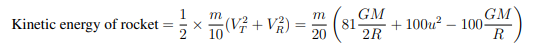=

$$\begin{array}{l}5m\left[u^{2}-\frac{119}{200}\frac{GM}{R}\right]\end{array}$$

15. Three point particles of mass 1 kg, 1.5 kg and 2.5 kg are placed at three corners of a right triangle of sides 4.0 cm, 3.0 cm and 5.0 cm as shown in the figure. The centre of mass of the system is at the point:
a. 0.9 cm right and 2.0 cm above 1 kg mass
b. 2.0 cm right and 0.9 cm above 1 kg mass
c. 1.5 cm right and 1.2 cm above 1 kg mass
d. 0.6 cm right and 2.0 cm above 1 kg mass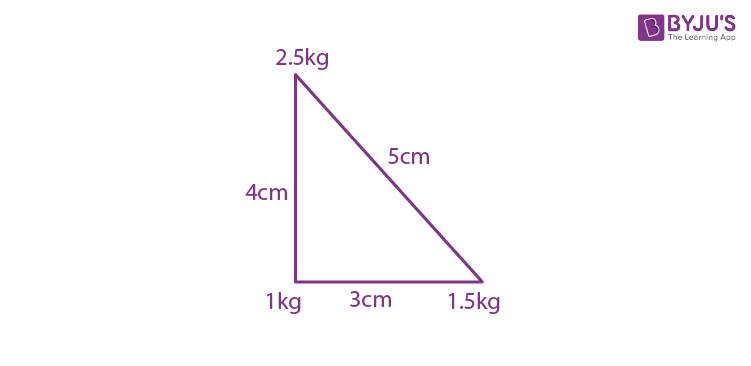Taking 1 kg as the origin

xcom= (m1x1 + m2x2 + m3x3)/(m1+m2+m3)

= (1 × 0 + 1.5 × 3 + 2.5 × 0)/5

xcom = 0.9

ycom= (m1y1 + m2y2 + m3y3)/ (m1 + m2+m3)

ycom= (1 × 0 + 1.5 × 0 + 2.5 × 4)/5

ycom = 2

Centre of mass is at (0.9, 2)

16. If we need a magnification of 375 from a compound microscope of tube length 150 mm and an objective of focal length 5 mm, the focal length of the eye-piece should be close to:
a. 22 mm
b. 12 mm
c. 2 mm
d. 33 mm

Magnification of compound microscope for least distance of distinct vision setting (strained eye)

M = (L/f0)(1 + (D/fe))

where L is the tube length

f0 is the focal length of objective

D is the least distance of distinct vision = 25 cm

$$\begin{array}{l}375 = \frac{150\times 10^{-3}}{5\times 10^{-3}}(1+\frac{25\times 10^{-2}}{f_{e}})\end{array}$$
$$\begin{array}{l}12.5 = (1+\frac{25\times 10^{-2}}{f_{e}})\end{array}$$
$$\begin{array}{l}\frac{25\times 10^{-2}}{f_{e}}=11.5\end{array}$$

∴ fe ≈ 21.7 × 10−3m = 22 mm

17. Speed of transverse wave on a straight wire (mass 6.0 g, length 60 cm and area of cross-section 1.0 mm2) is 90m/s. If the Young’s modulus of wire is 16 × 1011Nm−2, the extension of wire over its natural length is
a. 0.03 mm
b. 0.04 mm
c. 0.02 mm
d. 0.01 mm

Given, M = 6 grams = 6 × 10−3 kg

L = 60 cm = 0.6 m

A = 1 mm2 = 1 × 10−6m2

Using the relation, v2 = T/ µ

⇒ T = µv2

= V2 x M/L

As Young’s modulus, Y = stress/strain

strain = stress/Y= T/AY

strain =∆L/L = (V2(M/L))/AY = V2(M/AYL)

∆L = V2M/AY

∆L =(8100 × 6 × 10−3)/(1 × 10−6 × 16 × 1011)

∆L = 0.03 mm

18.1 liter of dry air at STP expands adiabatically to a volume of 3 litres. If γ = 1.4, the work done by air is (31.4 = 4.655) (take air to be an ideal gas)
a. 48 J
b. 100.8 J
c. 90.5 J
d. 60.7 J

Given, P1 = 1 atm, T1 = 273 K (At STP)

P1V1γ = P2V2γ

$$\begin{array}{l}P_{2}=P_{1}\left [ \frac{V_{1}}{V_{2}} \right ]^{\gamma }\end{array}$$

P2 = 1 × (1/3)1.4

P2 = 0.2164 atm

Work done in adiabatic process is given by

W =( P1V1 − P2V2)/(γ – 1)

W = ((1 × 1 − 3 × .2164)/ 0.4) x 101.325

Since, 1 atm = 101.325 kPa and 1 Liter = 10−3 m3

W=89.87J

19. A bob of mass m is tied by a massless string whose other end portion is wound on a fly wheel (disc) of radius r and mass m. When released from the rest, the bob starts falling vertically. When it has covered a distance h, the angular speed of the wheel will be:
a. r√3/4gh
b. (1/r)√4gh/3
c. ( r√3)/2gh
d. (1/r)√2gh/3

By energy conservation,

mgh = (1/2) mv2 + (1/2)Iω

⇒gh = v2/2 +ω2r2/4 ————(1)

Since the rope is inextensible and also it is not slipping,

∴ v = rω——–(2)

from eq. (1) and (2)

gh = ω2r2/2 +ω2r2/4

gh = (3/4) ω2r2

⇒ ω2 = 4gh/3r2

ω= (1/r) )√4gh/3

20. A parallel plate capacitor has plates of area A separated by distance ‘d’ between them. It is filled with a dielectric which has a dielectric constant varies as k (x) = k(1 + αx), where ‘x’ is the distance measured from one of the plates. If (αd <<1), the total capacitance of the system is best given by the expression:a.
$$\begin{array}{l}\frac{A\epsilon _{0}k}{d}\left [ 1+(\frac{\alpha d}{2})^{2}\right ]\end{array}$$

b.
$$\begin{array}{l}\frac{A\epsilon _{0}k}{d}\left [ 1+(\frac{\alpha d}{2})\right ]\end{array}$$

c.
$$\begin{array}{l}\frac{A\epsilon _{0}k}{d}\left [ 1+(\frac{\alpha^{2} d}{2})\right ]\end{array}$$

d.
$$\begin{array}{l}\frac{A\epsilon _{0}k}{d}\left [ 1+{\alpha d})\right ]\end{array}$$

Given, k(x) = k (1 + αx)

dC = Aε0k/dx

Since all capacitance are in series, we can apply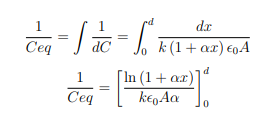On putting the limits from 0 to d

= ln (1 + αd)/ kε0

Using expression ln(1 + x) = x – x2/2 +—–

And putting x = αd where, x approaches to 0.

$$\begin{array}{l}\frac{1}{C}=\frac{d}{k\epsilon _{0}A\alpha }\left [ \alpha d-{\alpha^{2}d}^{2}/2)\right ]\end{array}$$
$$\begin{array}{l}C = \frac{A\epsilon _{0}k}{d\left ( 1-\frac{\alpha d}{2} \right )}\end{array}$$
$$\begin{array}{l}\Rightarrow C = \frac{\epsilon _{0}kA}{d}\left ( 1+\frac{\alpha d}{2} \right )\end{array}$$

21. A non- isotropic solid metal cube has coefficient of linear expansion as 5 × 10−5/C along the x-axis and 5 × 10−6/C along y-axis and z-axis. If the coefficient of volumetric expansion of the solid is C × 10−6/C then the value of C is

We know that, V = xyz

∆v/v = (∆x/x) + (∆y/y) + (∆z/z)

(1/T)∆v/v = (1/T)((∆x/x)+(∆y/y)+(∆z/z))

y = αn + αy + αz

y = (50 × 10−6/C) + (5 × 10−6/C) + (5 × 10−6/C)

y = 60 × 10−6/C

∴ C = 60

22. A loop ABCDEFA of straight edges has six corner points A(0,0,0), B(5,0,0), C(5,5,0), D(0,5,0), E(0,5,5), F(0,0,5). The magnetic field in this region is B˙ = (3ˆi + 4kˆ) T. The quantity of flux through the loop ABCDEFA (in Wb) is ———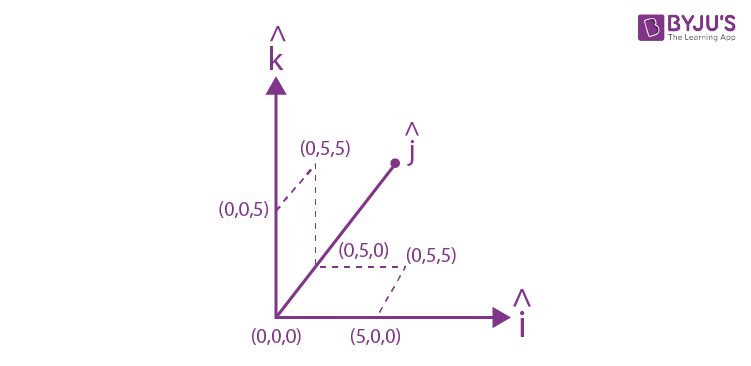As we know, magnetic flux =

$$\begin{array}{l}\vec{B}\times \vec{A}\end{array}$$

⇒ (Bx + Bz) • (Ax + Az)

⇒ (3ˆi + 4kˆ) • (25ˆi + 25kˆ)

⇒ (75 + 100) Wb

⇒ 175 Wb

23. A carnot engine operates between two reservoirs of temperature 900 K and 300 K. The engine performs 1200 J of work per cycle. The heat energy in(J ) delivered by the engine to the low temperature reservoir, in a cycle, is

η = 1 – (T2/T1) = 1- (300/900) =2/3

Given, W = 1200 J

From conservation of energy

Q1 − Q2 = W

η = Q1 − Q2 = W

⇒ Q1= 1800 J

⇒ Q2 = Q1 − W = 600 J

24. A particle of mass 1 kg slides down a frictionless track (AOC) starting from rest at a point A (height 2 m). After reaching C, the particle continues to move freely in air as a projectile. When it reaches its highest point P(height 1 m) the kinetic energy of the particle (in J ) is:
(Figure drawn is schematic and not to scale; take g = 10 m/s2)

As the particle starts from rest the total energy at point A = mgh = T.EA( where h = 2 m) After reaching point P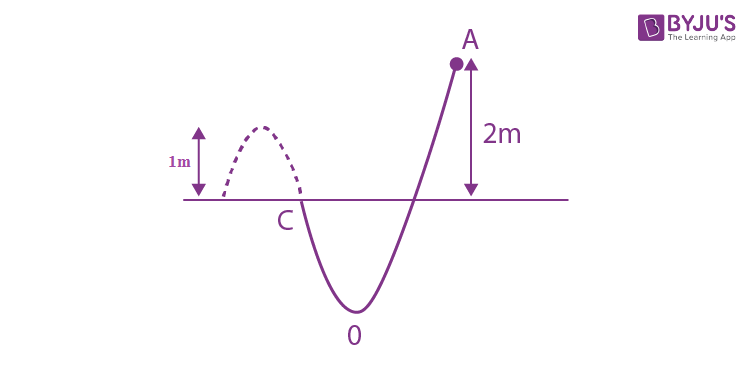T.Ec = K.E. + mgh

By conservation of energy

T.EA = T.Ep

⇒ K.E. = mgh = 10 J

25. A beam of electromagnetic radiation of intensity 6.4× 10−5 W/cm2 is comprised of wavelength, λ = 310 nm. It falls normally on a metal (work function φ = 2 eV) of surface area 1cm2. If one in 103 photons ejects an electron, total number of electrons ejected in 1s is 10x (hc = 1240 eV − nm, 1 eV = 1.6 × 10−19 J ), then x is

P = Intensity × Area

= 6.4 × 10−5Wcm−2 × 1 cm2

= 6.4 × 10−5 W

For photoelectric effect to take place, energy should be greater than work function Now,

E = (1240/310) = 4eV>2eV

Therefore, photoelectric effect takes place

Here n is the number of photons emitted.

n × E = I × A

n = IA/E = (6.4 × 10−5)/(6.4 × 10−19)

n = 1014

Where, n is number of incident photon

Since, 1 out of every 1000 photons are successful in ejecting 1 photoelectron Therefore, the number of photoelectrons emitted is

= 1014/103

x = 1011